Calculating Eigenvalues and Eigenvectors

Initialization Steps: Define a 2×2 matrix and compute its eigensystem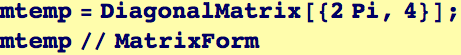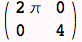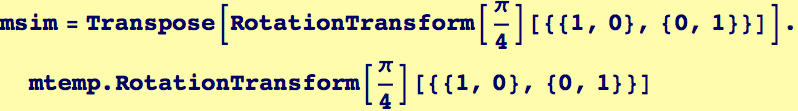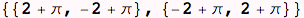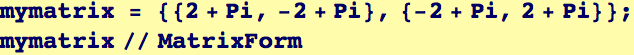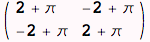Solve the characteristic equation for the two eigenvalues: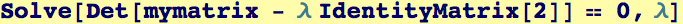Compute the eigenvectors: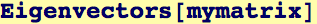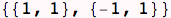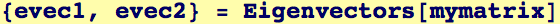Eigensystem will solve for eigenvalues and corresponding eigenvectors in one step: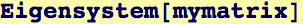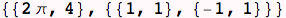Note the output format above: the first item in the list is a list of the two eigenvalues; the second item in the list is a list of the two corresponding eigenvectors.  Thus,  the eigenvector corresponding  2 π is (1,1).

 Created by Wolfram Mathematica 6.0  (12 September 2007)